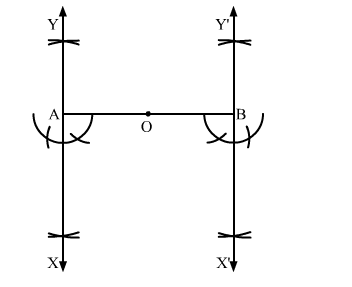# Draw a circle with centre O and radius 4 cm.`
Question:

Draw a circle with centre O and radius 4 cm. Draw any diameter AB of this circle. Construct tangents to the circle at each of the two end points of the diameter AB.

Solution:

Steps of Construction

Step 1. Draw a circle with centre O and radius 4 cm.

Step 2. Draw any diameter AOB of the circle.

Step 3. At A, draw ">OAX = 90º. Produce XA to Y.

Step 4. At B, draw ">OBX' = 90º. Produce X'B to Y'.Here, XAY and X'BY' are the tangents to the circle at the end points of the diameter AB.